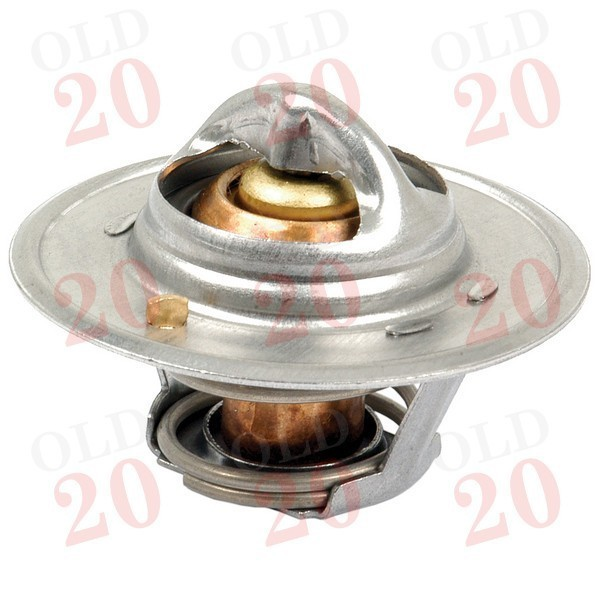View larger

# Thermostat

2053401

New

£ 7.20Tractors < Case IH < Vintage < 276
Tractors < Case IH < Vintage < 434
Tractors < Case IH < Vintage < 444
Tractors < Case IH < Vintage < B250
Tractors < Case IH < Vintage < B275
Tractors < Case IH < Vintage < B414
Tractors < Case IH < 94 < 1194
Tractors < Case IH < 94 < 1294
Tractors < Case IH < 94 < 1394
Tractors < Case IH < 94 < 1494
Tractors < Case IH < 94 < 1594
Tractors < Case IH < 94 < 1694
Tractors < David Brown < 94 < 1294
Tractors < David Brown < 94 < 1394
Tractors < David Brown < 94 < 1494
Tractors < David Brown < 94 < 1594
Tractors < David Brown < 94 < 1694
Tractors < Tractors < Ferguson < TEA20
Tractors < Tractors < Ferguson < TED20
Tractors < Tractors < Ferguson < TEF20
Tractors < Tractors < Ferguson < FE35 (87mm)
Tractors < Tractors < Ferguson < FE35 (23c)
Tractors < Leyland Marshall < Leyland < 245
Tractors < Leyland Marshall < Leyland < 253
Tractors < Leyland Marshall < Marshall < 502
Tractors < Massey Ferguson < Pre-100 Series < 35
Tractors < Massey Ferguson < Pre-100 Series < 35X
Tractors < Massey Ferguson < 100 < 135
Tractors < Massey Ferguson < 100 < 140
Tractors < Massey Ferguson < 100 < 145
Tractors < Massey Ferguson < 100 < 148
Tractors < Massey Ferguson < 100 < 152
Tractors < Massey Ferguson < 100 < 168
Tractors < Massey Ferguson < 100 < 175
Tractors < Massey Ferguson < 100 < 178
Tractors < Massey Ferguson < 100 < 185
Tractors < Massey Ferguson < 200 < 230
Tractors < Massey Ferguson < 200 < 240
Tractors < Massey Ferguson < 200 < 250
Tractors < Massey Ferguson < 200 < 254
Tractors < Massey Ferguson < 200 < 255
Tractors < Massey Ferguson < 200 < 265
Tractors < Massey Ferguson < 200 < 274
Tractors < Massey Ferguson < 200 < 275
Tractors < Massey Ferguson < 200 < 285
Tractors < Massey Ferguson < 200 < 290
Tractors < Massey Ferguson < 200 < 298
Tractors < Massey Ferguson < 300 < 340
Tractors < Massey Ferguson < 300 < 342
Tractors < Massey Ferguson < 300 < 350
Tractors < Massey Ferguson < 300 < 352
Tractors < Massey Ferguson < 300 < 353
Tractors < Massey Ferguson < 300 < 355
Tractors < Massey Ferguson < 300 < 360
Tractors < Massey Ferguson < 300 < 362
Tractors < Massey Ferguson < 300 < 377
Tractors < Massey Ferguson < 500 < 550
Tractors < Massey Ferguson < 500 < 565
Tractors < Massey Ferguson < 500 < 575
Tractors < Massey Ferguson < 500 < 590
Tractors < Massey Ferguson < 500 < 595
Tractors < Massey Ferguson < 500 < 595MKII
Tractors < Massey Ferguson < 600 < 675
Tractors < Massey Ferguson < 600 < 690
Tractors < Massey Ferguson < 600 < 698
Tractors < Massey Ferguson < 1000 < 1200

Supplier Reference :

 Section Cooling

Upto: 82°C

Diameter: 54mm

Height: 38.5mm